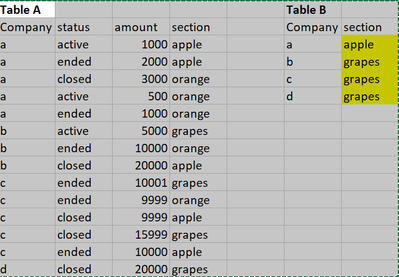cancel
Showing results for
Did you mean:Regular Visitor

## HELP: How to return text from related table but with order of conditions

Hi, I would like to return a text from a related table but with subsequent conditions: a) return text (section name) by following order of condition: active, ended, closed; if the company name has two active/ended/closed statuses, return the text (section name) with highest amount. See table A and B for reference.1 ACCEPTED SOLUTIONSolution Sage

One possible solution is as follows:

1) Create the following measure and make copies for Closed and Ended.

``````_Active =
VAR _Table1 =
FILTER(
'Table A',
'Table A'[status] = "active"
)
VAR _Result =
SWITCH(
COUNTROWS( _Table1 ),
0, BLANK(),
1, MAX( 'Table A'[section] ),
VAR _MaxValue =
MAXX(
_Table1,
'Table A'[amount]
)
RETURN
CALCULATE(
MAX( 'Table A'[section] ),
FILTER(
_Table1,
'Table A'[amount] = _MaxValue
)
)
)
RETURN
_Result``````

``zMeasure = COALESCE( [_Active], [_Ended], [_Closed] )``

pbix: speedcentaur.pbix

7 REPLIES 7Resolver I

Could you show an example of what you expect to see? Also could you copy and paste the 2 tables in a reply so I can work with them? 😎Regular Visitor

Hi. The table is pasted in my message. The highlighted in yellow in table 2 is what I want to achieve.Resolver I

Would I be correct in following this logic?

E.g...

1.) Look for company and if it has Status = Active return the section with the highest active value amount but if there is 2 or more active values return the highest value section?

2.) If however company doesn't have active status then move onto ended to get the ended value and return it's section but if there are 2 or more ended then return the highest ended section.

3.)Finally if the company doesn't have Active or ended then return closed section and if a company has 2 or more closed then return the highest closed section.

Just to be sure on the logic before I try to find a solution? 😊Regular Visitor

The logic is correct. Looking forward for the DAX solution. Thanks!Solution Sage

One possible solution is as follows:

1) Create the following measure and make copies for Closed and Ended.

``````_Active =
VAR _Table1 =
FILTER(
'Table A',
'Table A'[status] = "active"
)
VAR _Result =
SWITCH(
COUNTROWS( _Table1 ),
0, BLANK(),
1, MAX( 'Table A'[section] ),
VAR _MaxValue =
MAXX(
_Table1,
'Table A'[amount]
)
RETURN
CALCULATE(
MAX( 'Table A'[section] ),
FILTER(
_Table1,
'Table A'[amount] = _MaxValue
)
)
)
RETURN
_Result``````

``zMeasure = COALESCE( [_Active], [_Ended], [_Closed] )``

pbix: speedcentaur.pbixRegular Visitor

This is on point. Yes, that is the solution that I am looking for.Regular Visitor

@grantsamborn In addition to this measure, where I can add in this syntax to reflect "apple" first in the section. As there are instances that status and amount are exactly the same and I want to reflect "apple"

Thank you!